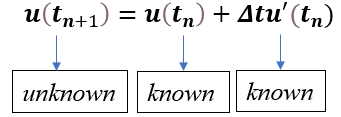top of page
Search

# Time integration Methods For Implicit And Explicit FEA - What Are They And How Do They Work?

The famous fourth dimension, ‘time’, plays a crucial role in determining the behavior of almost all engineering and physical systems. Given this, it is important that our FEA simulation methodologies account for time accordingly. This post is aimed at explaining the concept of time integration in FEA and the difference in how it is accounted for in implicit and explicit methods.

## Introduction

As we know, the motion of any physical system can be represented in terms of equation of motion. The general form of this equation of motion is,where is acceleration, is velocity and is deformation of the system. In the above equation, the acceleration, velocity, and deformation are unknowns with respect to time.

The acceleration and velocity are expressed in terms of deformation as,Hence, the equation of motion is second order ordinary differential equation in ’ with respect to time. This dynamic equation can either be solved in frequency domain or time domain. In frequency domain, the unknowns are assumed to be harmonic, and they have sinusoidal form. No such assumptions are made in the time domain and the equation of motion is directly solved for actual values of unknowns. But it is not always possible to find the continuous functions of the unknown parameters in the time domain. So, we have to divide the time into finite number of time steps and solve for the unknowns at this specific time points. This technique is called time integration method.In time integration technique, the unknown values in the current time step are calculated based on the known values for the previous time step. Based on computational process and applications, there are two types of time integration techniques: implicit and explicit. Let’s discuss the differences between these two techniques using displacement (u) and velocity (u’) equations at time step (tn+1) as shown below.The below graph shows the variation of u with respect to time t. tn and tn+1 are two consecutive time steps separated by time interval (∆t). The slope of the curve (u’) varies continuously with time (t).## Implicit Time Integration Method

In the implicit time integration method, the variables at unknown time step are calculated by using the slopes (u', u'') at unknown time step (tn+1). Since the displacement, velocity and acceleration are unknown at time step (tn+1), the equations are not solved directly.So, in implicit method we will get a set of equations with unknown variables at different time steps which have to be solved simultaneously. In general, these equations are not linear. We have to use numerical methods like Newton- Raphson, secant method, inverse quadratic interpolation etc., to solve for the converged unknown quantities. These numerical methods are computationally very expensive. But the implicit method gives stable solution irrespective of time step. Hence this method is unconditionally stable.

## Explicit Time Integration Method

In explicit method, we use the slopes (u', u'') at known time step to calculate the variables at unknow time step (tn+1). Since the displacement, velocity and acceleration are known at time step (tn), the equations are solved directly.Since the unknown values are calculated directly, numerical methods are not needed in explicit process. Because of the forward moving nature of the explicit method, it requires small time increments.

The size of the time step is determined such that the dilation wave cannot travel more than the smallest characteristic length of the element. This is termed as Courant-Friedrichs-Lewy (CFL) condition. This is mathematically expressed as,where Lmin is the smallest element dimension in the mesh and cd is the longitudinal dilation wave speed. We consider the speed of longitudinal wave here as it travels faster than the transverse wave. Since it’s the smallest element dimension determining the time step size in explicit method, it is recommended to have uniform elements in the model to avoid small time steps due to one or few small elements.

If we don’t know the dilation wave speed, it can be calculated using material properties as follows,where E is young’s modulus and ρ is the density of the material.

This calculated time step might be too small in large FEA models and the simulation might take very long time to complete. From the above equations, we see that time step size is inversely proportion to wave speed. This means that if we can artificially decrease the wave speed, the time step size can be increased. The wave speed can be decreased by artificially increasing the density of the material. This method of artificially increasing the times step size by increasing the density of the material is called Mass scaling. However, care should be taken to apply mass scaling to only set of smallest elements in the model to preserve the global accuracy of the results.

The table below provides a summary of the differences between implicit and explicit time integration techniques.## Final Thoughts

Time integration methods play a crucial role in solving partial differential equations in FEA. Hopefully this article has provided some basic understanding of explicit and implicit time integration methods and their working.

If you need help with FEA or simulation in general, don’t hesitate to give us a call! We’re passionate about what we do, and we would love to learn more about your unique engineering challenges.

2,293 views

See All
bottom of page